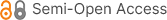Number Theory and Algebraic Equations

ISBN: 978-1-940366-74-6
Published Date: November, 2016
Pages: 240
Paperback: \$120
E-book: \$25
Publisher: Science Publishing Group
 To purchase hard copies of this book, please email: book@sciencepublishinggroup.com
Book Description

The book covers the classical number theory of the 17-19th centuries with simple algebraic proofs: theorems published by Fermat (his Last Theorem), Euler, Wilson, Diophantine equations, Lagrange and Legendre Theorems on the representation of integers as sums of squares and other classes of numbers, the factorization of polynomials, Catalan’s and Pell’s equations. It provides proofs of several conjectures and new generalizations of the classical theory, with applications to the prime numbers and polynomials.

A second part concerns the expansions in series of functions related to the cardinal of the primes, the functions Gamma and zeta. Several known formulas and new results are proved.

A third part introduces the algebra of rings and fields in relation to the theory the polynomials and Galois’s theory, which are applied to the resolution of algebraic equations. The classical theorems are extended to polynomials and to the complex field. Numerical examples and approximately 50 exercises with proofs illustrate the main topics.

Author Introduction

Odile Marie-Thérèse Pons is a Senior Research Fellow in Mathematics at the French National Institute for Agronomical Research. She holds MSc., doctor’s degree and habilitation qualification in Mathematics at the university of Paris. She is the author of about a hundred of peer-reviewed articles in mathematical and applied statistics and probability, and 8 books in Probability, Statistics and Analysis.

• The Whole Book

• Front Matter

• Chapter 1 Introduction

1. 1.1 Factorization of the Integers
2. 1.2 Polygonal Numbers
5. 1.5 Exercises
• Chapter 2 Fermat’s First Theorem and Quadratic Residues

1. 2.1 Fermat’s First Theorem
2. 2.2 Divisors of an Integer
4. 2.4 Wilson’s Theorem and Sums of Squares
5. 2.5 Euler’s φ(n)
6. 2.6 Exercises
• Chapter 3 Algebraic Equations and Fermat’s Last Theorem

1. 3.1 Algebraic Equations
2. 3.2 Fermat’s Last Theorem
3. 3.3 Catalan’s Equation
4. 3.4 Generalizations of Fermat’s Last Theorem
5. 3.5 Exercises
• Chapter 4 Prime Numbers and Irrational Numbers

1. 4.1 Cardinal of the Primes in Intervals
4. 4.4 Algebraic Numbers of Degree n
5. 4.5 Equations with Several Variables
6. 4.6 Twin-Primes
7. 4.7 Exercises
• Chapter 5 Euler’s Functions

1. 5.1 Definition
2. 5.2 Approximations of the Function π (x)
3. 5.3 Approximations of the Function Gamma
4. 5.4 Riemann’s Equations for ζs
5. 5.5 The Alternating Series
6. 5.6 Bernoulli Polynomials
7. 5.7 Trigonomeric Expansions
8. 5.8 The Hurwitz Zeta Function
9. 5.9 Logarithm of ζs
10. 5.10 Exercises
• Chapter 6 Automorphisms

1. 6.1 Rings
2. 6.2 Algebraic Extension of a Field
3. 6.3 Galois’s Extension
4. 6.4 Galois’s Theory
5. 6.5 Equation Solvable by Radicals
6. 6.6 Exercises
• Chapter 7 Functional Equations

1. 7.1 Fermat's First Theorem for Polynomials
2. 7.2 Polynomials in Fp
3. 7.3 Quadratic Equations for Polynomials
4. 7.4 Complex Algebraic Fields
5. 7.5 Exercises
• Chapter 8 Solutions of the Exercises

1. 8.1 Introduction
2. 8.2 Fermat’s First Theorem and Quadratic Residues
3. 8.3 Algebraic Equations and Fermat’s Last Theorem
4. 8.4 Prime Numbers and Irrational Numbers
5. 8.5 The Function Zeta
6. 8.6 Automorphisms
7. 8.7 Functional Equations
• Back Matter